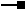# IRasterColormap4 Interface

## Members

Name DescriptionBin Translates pixel values into integers to index them into the colormap.BinFunction The Bin function that maps pixel value into colormap index.BlueValues Array of blue ratios as doubles between 0.0 and 1.0.Colors Array of colors as OLE_​COLORs.GreenValues Array of green ratios as doubles between 0.0 and 1.0.LoadFrom Loads colors from an Esri .clr file.LoadFromString Loads from a colormap definition string.LUT The lookup table (OBJECTID, VALUE) for remaping from index to pixel value.QueryMinMax Queries minimum and maximum pixel values.RedValues Array of red ratios as doubles between 0.0 and 1.0.RemappedBlueValues The remapped Blue color component values using LUT from index to value (OBJECTID, VALUE).RemappedColors The remapped colors using LUT from index to value (OBJECTID, VALUE).RemappedGreenValues The remapped Green color component values using LUT from index to value (OBJECTID, VALUE).RemappedRedValues The remapped Red color component values using LUT from index to value (OBJECTID, VALUE).SaveTo Saves colors to an Esri .clr file.Size The number of colors in this colormap.Values The list of pixel values.

Loads from a colormap definition string.

``````Public Sub LoadFromString ( _
ByVal colormapDefinition As String _
)
``````
``````public void LoadFromString (
string colormapDefinition
);
``````

### IRasterColormap4.QueryMinMax Method

Queries minimum and maximum pixel values.

``````Public Sub QueryMinMax ( _
ByRef Min As Integer, _
ByRef Max As Integer _
)
``````
``````public void QueryMinMax (
ref int Min,
ref int Max
);
``````

### IRasterColormap4.Size Property

The number of colors in this colormap.

``````Public ReadOnly Property Size As Integer
``````
``````public int Size {get;}
``````

### IRasterColormap4.Values Property

The list of pixel values.

``````Public Property Values As Object
``````
``````public object Values {get; set;}
``````

## Inherited Interfaces

Interfaces Description